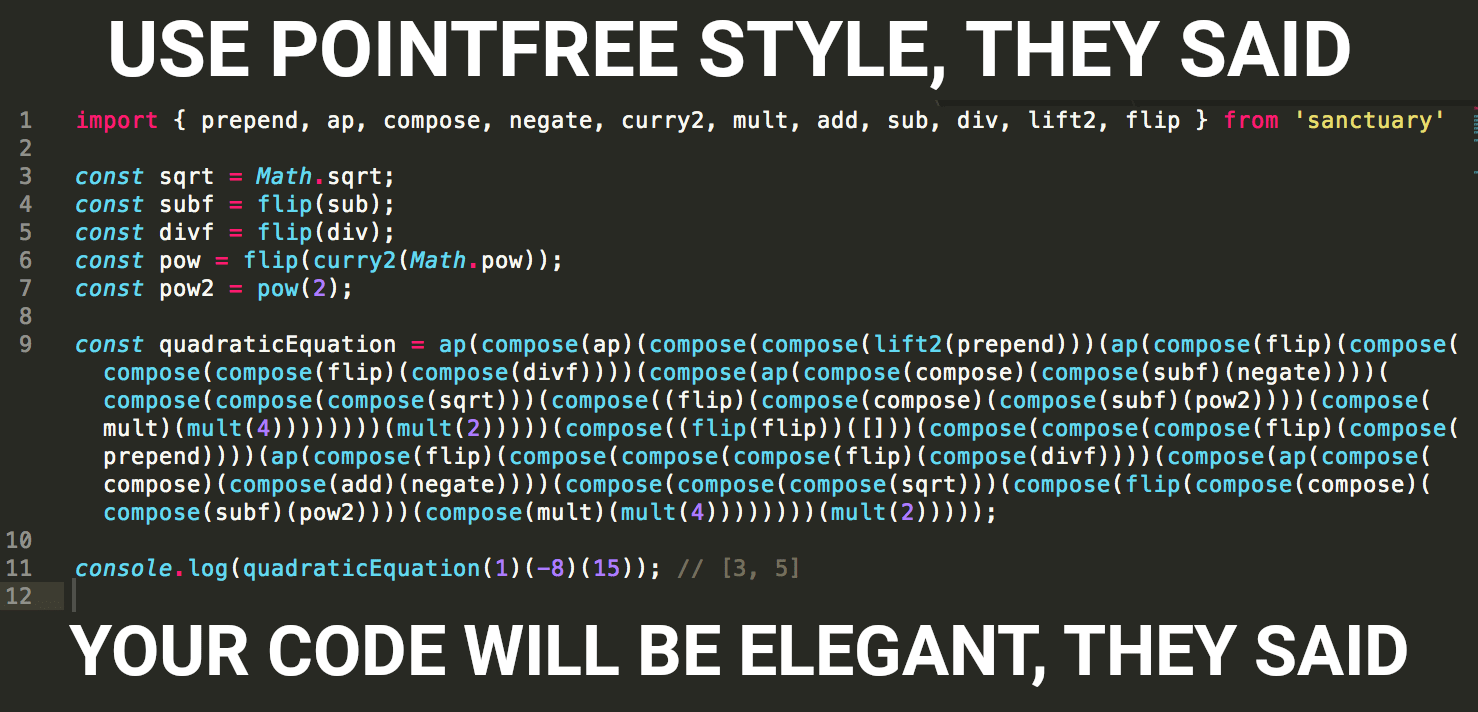Switch branches/tags
Nothing to show

A tag already exists with the provided branch name. Many Git commands accept both tag and branch names, so creating this branch may cause unexpected behavior. Are you sure you want to create this branch?

## Files

Failed to load latest commit information.
Type
Name
Commit time# Using Haskell magic for JavaScript

Actually, you don't need it.

This is annswer for question here: `https://www.reddit.com/r/ProgrammerHumor/comments/a3d30v/go_functional/`

Initially, I just wanted to show that you need to think twice before using pointfree form. First time about 5-10 minutes about rewrite pointwise function in pointfree form and second time (as much as you need) about not to do it if you didn't find pointfree form fast enough. To show it I found magic method how to make your colleagues want to kill you.
During this researching I found some problem in Ramda. I created issue and Scott Sauyet wrote me function to demonstrate why it's not a bug in Ramda ( ramda/ramda#2731 ).
This function:

```const quadraticEquation = (a, b, c) => [
(-b - Math.sqrt(b * b - 4 * a * c)) / (2 * a),
(-b + Math.sqrt(b * b - 4 * a * c)) / (2 * a),
] // e.g. quadraticEquation(1, -8, 15) //=> [3, 5]```

Then I just applyed method I found earlier for this function to create meme.

1. I wrote it in Haskell form:
``````\a b c -> [(-b - sqrt(b * b - 4 * a * c)) / (2 * a), (-b + sqrt(b * b - 4 * a * c)) / (2 * a)]
``````

To check in GHCi:

``````(\a b c -> [(-b - sqrt(b * b - 4 * a * c)) / (2 * a), (-b + sqrt(b * b - 4 * a * c)) / (2 * a)]) 1 (-8) 15
``````

I think it looks a lot better than in JavaScript form.

1. There is special tool in Haskell that allow to refactor pointwise to pointfree: http://hackage.haskell.org/package/pointfree It's open-source, so you can try to understand how it work.
For this tool you can use web interface: http://pointfree.io/ It converts this function into:
`ap (ap . (liftM2 (:) .) . ap (flip . ((flip . ((/) .)) .) . ap ((.) . (-) . negate) . ((sqrt .) .) . flip ((.) . (-) . join (*)) . (*) . (4 *)) (2 *)) (flip flip ([]) . ((flip . ((:) .)) .) . ap (flip . ((flip . ((/) .)) .) . ap ((.) . (+) . negate) . ((sqrt .) .) . flip ((.) . (-) . join (*)) . (*) . (4 *)) (2 *))`

In GHCi:

`(ap (ap . (liftM2 (:) .) . ap (flip . ((flip . ((/) .)) .) . ap ((.) . (-) . negate) . ((sqrt .) .) . flip ((.) . (-) . join (*)) . (*) . (4 *)) (2 *)) (flip flip ([]) . ((flip . ((:) .)) .) . ap (flip . ((flip . ((/) .)) .) . ap ((.) . (+) . negate) . ((sqrt .) .) . flip ((.) . (-) . join (*)) . (*) . (4 *)) (2 *))) 1 (-8) 15`
1. Haskell syntax a lot better for curried functions. So I need to uglify it to be able to convert in JavaScript:
`ap ((.) ap ((.) ((.) (liftM2 (:))) (ap ((.) flip ((.) ((.) ((.) flip ((.) (/)))) ((.) (ap ((.) (.) ((.) (-) negate))) ((.) ((.) ((.) sqrt)) ((.) (flip ((.) (.) ((.) (-) (join (*))))) ((.) (*) ((*) 4))))))) ((*) 2)))) ((.) ((flip flip) ([])) ((.) ((.) ((.) flip ((.) (:)))) (ap ((.) flip ((.) ((.) ((.) flip ((.) (/)))) ((.) (ap ((.) (.) ((.) (+) negate))) ((.) ((.) ((.) sqrt)) ((.) (flip ((.) (.) ((.) (-) (join (*))))) ((.) (*) ((*) 4))))))) ((*) 2))))`

In GHCi:

`(ap ((.) ap ((.) ((.) (liftM2 (:))) (ap ((.) flip ((.) ((.) ((.) flip ((.) (/)))) ((.) (ap ((.) (.) ((.) (-) negate))) ((.) ((.) ((.) sqrt)) ((.) (flip ((.) (.) ((.) (-) (join (*))))) ((.) (*) ((*) 4))))))) ((*) 2)))) ((.) ((flip flip) ([])) ((.) ((.) ((.) flip ((.) (:)))) (ap ((.) flip ((.) ((.) ((.) flip ((.) (/)))) ((.) (ap ((.) (.) ((.) (+) negate))) ((.) ((.) ((.) sqrt)) ((.) (flip ((.) (.) ((.) (-) (join (*))))) ((.) (*) ((*) 4))))))) ((*) 2))))) 1 (-8) 15`

Now we are ready to convert it to JavaScript.

1. You can use Ramda (https://ramdajs.com/) or Sanctuary (https://sanctuary.js.org/) if you don't want to build all these functional programming functions. I did it for both to find what I like more for future use. Looks like, I Sanctuary is my choice for next projects. But I build https://battleship-fp.com/ , when I wanted to try functional programming and Haskell in real world project, with Ramda.
So for Ramda I have ( https://codesandbox.io/s/5w2wjq8x9n ):
```import { prepend, ap, compose, negate, curryN, flatten, multiply, add, subtract, divide, lift } from 'ramda'
const flip = f => x => y => f(y)(x);
const cc2 = curryN(2)(compose);
const sqrt = Math.sqrt;
const pow = flip(curryN(2)(Math.pow));
const pow2 = pow(2);

And for Sanctuary ( https://codesandbox.io/s/n45rlor7j4 ):

```import { prepend, ap, compose, negate, curry2, mult, add, sub, div, lift2, flip } from 'sanctuary'

const sqrt = Math.sqrt;
const subf = flip(sub);
const divf = flip(div);
const pow = flip(curry2(Math.pow));
const pow2 = pow(2);

That's it.
Now you can to blow your colleagues mind off with tricky functions. Please, don't do it. :)

BTW, if someone need good developer, I'll be happy to discuss it. Especially if you are using Functional Programming for your projects.

No description, website, or topics provided.

## Releases

No releases published

## Packages 0

No packages published